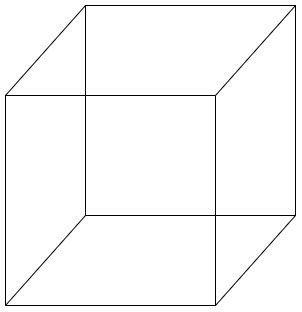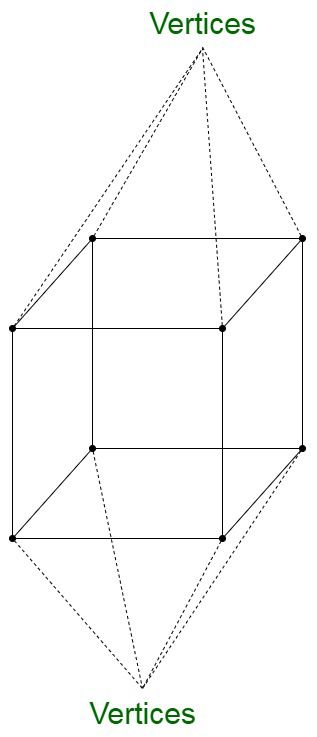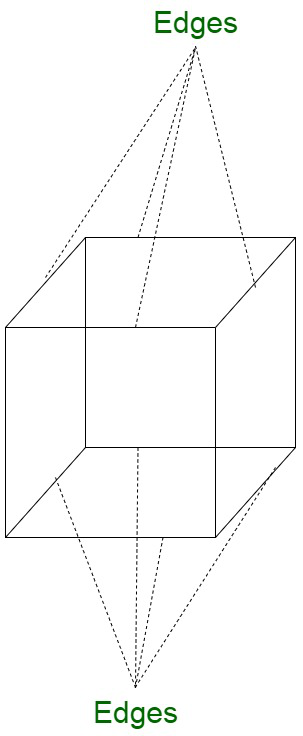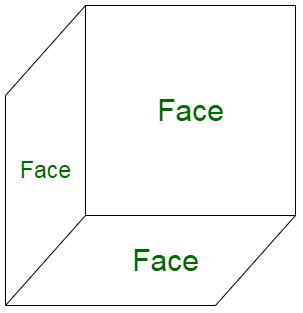GeeksforGeeks App
Open AppBrowser
Continue

# How many faces, edges, and vertices does a cube have?

Mensuration is a division of mathematics that deals with the study of various available geometrical figures and their associated parameters such as area, length, volume, lateral surface area, surface area, etc.

Cube

A cube can be visualized in the form of a square prism. This is because the faces of a cube are in the form of a square and are also platonic solid in nature. The faces of a cube are also known as planes.Properties of a Cube

• All the faces are square-shaped, which implies that the length, breadth, and height are the same.
• The angles between any two faces or surfaces are equivalent to 90°.
• The opposite planes are parallel to each other.
• The opposite edges are parallel to each other.
• Each of the faces forms intersection with four faces.
• Each of the vertices intersects with three faces and three edges.

### Vertices of cube

A vertex of any figure is a point where two or more line segments form an intersection. It can be considered as a corner. There are 8 vertices in a cube.### Edges of cube

An edge in any geometrical figure can be considered as a line segment where any two faces form an intersection. They form the skeleton of the 3D shapes. It forms a boundary joining one vertex (corner point) to another. A cube has 12 edges.### Faces of cube

The face of any geometrical figure is the individual flat surfaces of a solid object. A cube has 6 faces.Formula of cube

• Surface Area of Cube = 6a2 in square units

Where, ‘a’ is the side of the cube

• Volume of cube = a3 cubic units

Where, ‘a’ is the side of the cube

### Sample Questions

Question 1. Find the surface area of the cube if its side is 6 cm?

Solution:

Here we need to find the surface area of the cube.

Given:

Side of the cube = 6 cm

As we know that

Surface area of the cube = 6 × side × side

Surface area of the cube = 6 × side2

Surface area of the cube = 6 × 62

Surface area of the cube = 216 cm2

Therefore,

Surface area of the cube is 216 cm2.

Question 2. Find the volume of the cube if its side is 4 m2?

Solution:

Here we need to find the volume of the cube

Given:

Side of the cube = 4 m2

As we know that

Volume of the cube = Side × Side × Side

Volume of the cube = Side3

Volume of the cube = 43

Volume of the cube = 4 × 4 × 4

Volume of the cube = 64 m3

Therefore,

Volume of the cube is 64 m3.

Question 3. Find how many small cubes can be made from a big cube of side 16 m in small cubes of side 4 m?

Solution:

Here we need to find out how many small cubes can be made out of one big cube.

As we know that

Volume of cube = Side3

Volume of big cube = Side × Side × Side

Volume of big cube = 16 × 16 × 16

Volume of big cube = 163

Volume of big cube = 4096 m3

Further,

Volume of small cube = Side × Side × Side

Volume of small cube = 4 × 4 × 4

Volume of small cube = 43

Volume of small cube = 64 m3

Now,

Number of small cubes that can be made from the big cubes = Volume of big cube/Volume of small cube

Number of small cubes = 4096/64

Number of small cubes = 64

Therefore,

64 small cubes will be made out of the big cube.

Question 4. If the surface area of a cube is 486 m2. Then find the volume of the cube?

Solution:

Here we need to find the volume of the cube from a given surface area

Given:

Surface area of the cube = 486 m2

As we know that

Surface area of the cube = 6 × Side2

486 = 6 × Side2

Side2 = 486/6

Side2 =  81

Side = √81

Side = 9 m

Now,

Volume of cube = Side3

Volume of cube = 93

Volume of cube = 9 × 9 × 9

Volume of cube = 729 m3

Therefore,

Volume of the cube is 729 m3.

My Personal Notes arrow_drop_up
Related Tutorials